# 4. Spheres as manifolds¶

This notebook is part of the Introduction to manifolds in SageMath by Andrzej Chrzeszczyk (Jan Kochanowski University of Kielce, Poland).

In :
version()

Out:
'SageMath version 9.6, Release Date: 2022-05-15'

## One dimensional sphere $\mathbf{S}^1$¶

Example 4.1

In manifolds.Sphere in the one-dimensional case $\ \ S^1=\{(x,y)\in R^2: x^2+y^2=1\}\ \$ the default coordinates are the spherical (polar) ones.

In :
%display latex
S1=manifolds.Sphere(1)                      # 1-dimensional sphere
Phi=S1.embedding()                          # embedding S^1 -> E^2
Phi.disp()                                  # show embedding

Out:
$\displaystyle \begin{array}{llcl} \iota:& \mathbb{S}^{1} & \longrightarrow & \mathbb{E}^{2} \\ \mbox{on}\ A : & \phi & \longmapsto & \left(x, y\right) = \left(\cos\left(\phi\right), \sin\left(\phi\right)\right) \end{array}$

The spherical coordinates are predefined in manifolds.Sphere but we want to have a shorter name.

In :
sph.<phi>=S1.spherical_coordinates()       # spherical coordinates
sph.coord_range()                          # coordinate range

Out:
$\displaystyle \phi :\ \left[ -\pi , \pi \right] \mbox{(periodic)}$

On $\ [-\pi,\pi]\$ the map $\varphi \to (\cos\varphi, \sin\varphi)$ is not one-to one, so not homeomorphic.

To represent graphically the sphere $S^1$ we need the ambient space $R^2$ and the mapping $S^1 \to R^2$.

In :
E=S1.ambient()                            # ambient space E^2
c_cart.<x,y> = E.cartesian_coordinates()  # Cartesian coord in R^2
p=sph.plot(c_cart,mapping=Phi,number_values=15,
thickness=1,color='grey')             # plot S^1
p.show(figsize=[3,2])                     # show plot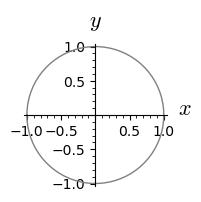Restricting the coordinate to an open interval contained in $[-\pi,\pi]$ we obtain a homeomorphic map (but the image is a proper subset of the sphere).

In :
p=sph.plot(c_cart,mapping=Phi,ranges={phi:(-pi,pi/4)},
number_values={phi:50},color={phi:'grey'})  # plot subset of S^1
p.show(figsize=[3,2])                         # show plot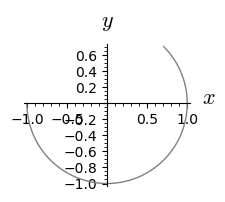Example 4.2

$S^1$ can be defined as a manifold with two-element atlas. Note that in this new example we do not use the manifolds.Sphere command!

In :
# example from the Manifolds manual, sect 2.7.1 (p.546 in 9.5 version)
M = Manifold(1, 'S^1')          # manifold S^1
U = M.open_subset('U')          # the complement of one point (1,0)
c_u.<u> = U.chart('u:(0,2*pi)') # the standard angle coordinate
V = M.open_subset('V')          # the complement of the point (-1,0)
c_v.<v> = V.chart('v:(0,2*pi)') # the angle u-pi
M.declare_union(U,V)            # S^1 is the union of U and V
M.atlas()                       # atlas

Out:
$\displaystyle \left[\left(U,(u)\right), \left(V,(v)\right)\right]$

On the intersection of $W=U\cap V$ two "new" charts are defined, they are just restrictions of the "old" maps to $W$.

In :
u_to_v = c_u.transition_map(c_v, (u-pi,), # transition map u->v
intersection_name='W',
restrictions1 = u!=0,
restrictions2 = v!=pi)
v_to_u = u_to_v.inverse()                 # inverse transition
M.atlas()                                 # atlas

Out:
$\displaystyle \left[\left(U,(u)\right), \left(V,(v)\right), \left(W,(u)\right), \left(W,(v)\right)\right]$

If we need to use $W=U\cap V$ in calculations we can define it:

In :
W = U.intersection(V)                    # intersection of
print(W)                                 # chart domains

Open subset W of the 1-dimensional differentiable manifold S^1


To make the plot in $R^2$ we need to define an ambient space and the embedding.

In :
R2 = Manifold(2, 'R^2')                 # manifold R^2
X.<x,y> = R2.chart()                    # Cartesian coordinates
# the embedding is not predefined this time, so it must be
# defined:
F = M.continuous_map(R2, {(c_u, X): [cos(u),sin(u)]}, name='F')
p=c_u.plot(X,mapping=F,color='grey')    # plot image of S^1
p.show(figsize=(3,2))                   # show plot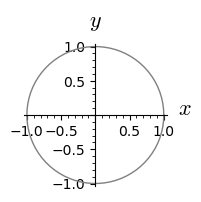### Stereographic coordinates on $\mathbf{S}^1$¶

Example 4.3

In manifold.Sphere not only the Cartesian and polar but also the stereographic coordinates are predefined.

In :
S1=manifolds.Sphere(1)         # stereographic projection from the
S1.stereographic_coordinates(pole='north',names='u')  # North pole

Out:
$\displaystyle \left(\mathbb{S}^{1}\setminus\{\mathrm{NP}\},(u)\right)$
In :
                               # stereographic projection from the
S1.stereographic_coordinates(pole='south',names='u')  # South pole

Out:
$\displaystyle \left(\mathbb{S}^{1}\setminus\{\mathrm{SP}\},({u'})\right)$

### Stereographic projection of $S^1$ from the North pole $N = (0, 1)$¶

In :
var('t')                                        # symb. variable
p1=parametric_plot((cos(t),sin(t)),(t,0,2*pi),color='grey')
# plot the circle
p2=plot(1-x/2,(x,0,2))          # half line through (0,1) and (x,y)
p3= text("(x,y)",(0.88,0.72))                   # point (x,y)
p4= text("(u,0)",(2.0,0.15))                    # point (u,0)
(p1+p2+p3+p4).show(figsize=[3,2])               # combine plots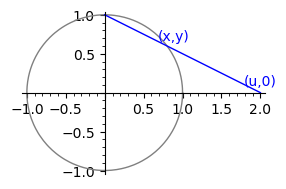Consider the line which passes through the North pole $(0, 1)$, the point $(x, y)$ on the circle and intersects the axis Ox at the point $(u, 0)$. The function $u = u(x, y)$ defines the stereographic projection. Three points: North pole, the point $(x, y)$ on the circle and the point $(u, 0)$ are on the same line. Since the right triangle with the hypotenuse joining (0,1), (x,y) and the right triangle with the hypotenuse joining (0,1),(u,0) are similar, we have $\frac{u}{x}=\frac{1}{1-y}$ and consequently $u=\frac{x}{1-y}$. Solving the system of equations $$x^2+y^2=1,\quad u=\frac{x}{1-y},$$ with respect to $(x,y)$:

In :
%display latex
forget()                               # forget previous assumptions
var('x, y, u')                               # symbolic variables
assume(y>0)                                  # assume y>0
s=solve([x^2+y^2==1,u==x/(1-y)],[x,y]);s     # solve eq. above

Out:
$\displaystyle \left[\left[x = \frac{2 \, u}{u^{2} + 1}, y = \frac{u^{2} - 1}{u^{2} + 1}\right], \left[x = 0, y = 1\right]\right]$

we obtain the relations $$x=\frac{2u}{u^2+1},\quad y=\frac{u^2-1}{u^2+1}, \quad u=\frac{x}{1-y}.$$

Now consider the South pole case.

### Stereographic projection of $S^1$ from the South pole $S = (0, -1)$¶

In :
var('t')                                       # symbolic variable
p1=parametric_plot((cos(t),sin(t)),(t,0,2*pi),color='grey')
# plot the circle
p2=plot(x/2-1,(x,0,2))          # halfline through (0,-1) and (x,y)
p3= text("(x,y)",(0.88,-0.72))                 # point (x,y)
p4= text("(u',0)",(2.0,0.15))                  # point (u',0)
(p1+p2+p3+p4).show(figsize=[3,2])              # combine plots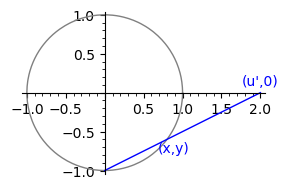Since the right triangle with the hypotenuse joining (0,-1), (x,y) and the right triangle with the hypotenuse joining (0,-1),(u',0) are similar we have $\frac{u'}{x}=\frac{1}{1-(-y)}$ ($y$ is negative now) and consequently $u'=\frac{x}{1+y}$. Solving the system of equations $$x^2+y^2=1,\quad u'=\frac{x}{1+y},$$ with respect to $(x,y)$:

In :
forget()                               # forget previous assumptions
var('x, y, up')                               # symbolic variables
assume(y<0)                                   # assume y<0
s=solve([x^2+y^2==1,up==x/(1+y)],[x,y]);s     # solve eq. above

Out:
$\displaystyle \left[\left[x = \frac{2 \, {u'}}{{u'}^{2} + 1}, y = -\frac{{u'}^{2} - 1}{{u'}^{2} + 1}\right], \left[x = 0, y = \left(-1\right)\right]\right]$

we obtain the relations $$x=\frac{2u'}{u'^2+1},\quad y=\frac{1-u'^2}{u'^2+1}, \quad u'=\frac{x}{1+y}.$$

Example 4.4

As we mentioned, both the stereographic projections from North and South poles are predefined in manifolds.Sphere.

In :
reset()
S1=manifolds.Sphere(1)                    # S^1 from manifolds.Sphere
# stereographic projection from the North pole:
stereoN.<u> = S1.stereographic_coordinates(pole='north')
# stereographic projection from the South pole:
stereoS.<up> = S1.stereographic_coordinates(pole='south')

Phi=S1.embedding()                        # embedding S^1 -> E^2
Phi.disp()                                # show embedding

Out:
$\displaystyle \begin{array}{llcl} \iota:& \mathbb{S}^{1} & \longrightarrow & \mathbb{E}^{2} \\ \mbox{on}\ A : & \phi & \longmapsto & \left(x, y\right) = \left(\cos\left(\phi\right), \sin\left(\phi\right)\right) \\ \mbox{on}\ \mathbb{S}^{1}\setminus\{\mathrm{NP}\} : & u & \longmapsto & \left(x, y\right) = \left(\frac{2 \, u}{u^{2} + 1}, \frac{u^{2} - 1}{u^{2} + 1}\right) \\ \mbox{on}\ \mathbb{S}^{1}\setminus\{\mathrm{SP}\} : & {u'} & \longmapsto & \left(x, y\right) = \left(\frac{2 \, {u'}}{{u'}^{2} + 1}, -\frac{{u'}^{2} - 1}{{u'}^{2} + 1}\right) \end{array}$

Example 4.5

To show graphically how the projection from the North pole acts, let us extract an appropriate part of the definition from the previous cell.

In :
# continuation
E=S1.ambient()                                    # ambient space E^2
c_cart.<x,y> = E.cartesian_coordinates()          # Cartesian coord.
fun=list(Phi.coord_functions(stereoN).expr())     # embedd. functions
PhiN = S1.continuous_map(E,{(stereoN, c_cart): fun},
name='PhiN',latex_name=r'\Phi_N')         # define embedding
PhiN.display()                                    # show embedding

Out:
$\displaystyle \begin{array}{llcl} \Phi_N:& \mathbb{S}^{1} & \longrightarrow & \mathbb{E}^{2} \\ \mbox{on}\ \mathbb{S}^{1}\setminus\{\mathrm{NP}\} : & u & \longmapsto & \left(x, y\right) = \left(\frac{2 \, u}{u^{2} + 1}, \frac{u^{2} - 1}{u^{2} + 1}\right) \end{array}$

Let us plot the set of points $(x,y)$ corresponding to $u\in (-10,10)$.

In :
p=stereoN.plot(c_cart,mapping=PhiN,ranges={u:(-10,10)},
number_values={u:50},color={u:'grey'},
plot_points=500)                  # image of (-10,10) under PhiN
p.show(figsize=[3,2])               # show the image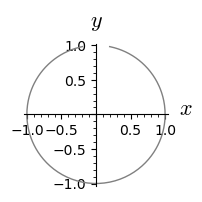Thus the points of the grey arc in the figure are projected onto the interval (-10,10).

Example 4.6

Now let us extract the definition of the projection from the South pole,

In :
# continuation
fun=list(Phi.coord_functions(stereoS).expr())    # embedd. functions
PhiS = S1.continuous_map(E,{(stereoS, c_cart): fun},
name='PhiS',latex_name=r'\Phi_S')            # define embedding
PhiS.display()                                   # show embedding

Out:
$\displaystyle \begin{array}{llcl} \Phi_S:& \mathbb{S}^{1} & \longrightarrow & \mathbb{E}^{2} \\ \mbox{on}\ \mathbb{S}^{1}\setminus\{\mathrm{SP}\} : & {u'} & \longmapsto & \left(x, y\right) = \left(\frac{2 \, {u'}}{{u'}^{2} + 1}, -\frac{{u'}^{2} - 1}{{u'}^{2} + 1}\right) \end{array}$

and check which points of the circle are projected onto (-10,10):

In :
# continuation
p=stereoS.plot(c_cart,mapping=PhiS,ranges={up:(-10,10)},
number_values={up:50},color={up:'grey'},   # plot image of (-10,10)
plot_points=500)
p.show(figsize=[3,2])                        # show image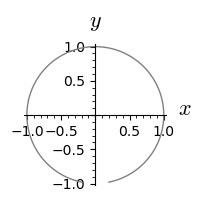### Transition map from u coordinate to u' coordinate¶

Using the relations $\ u=\frac{x}{1-y},\ \ u'=\frac{x}{1+y}$ and $y=\frac{u^2-1}{u^2+1},\$ we obtain $u'=\frac{x}{1+y}=\frac{u(1-y)}{1+y}=\frac{1-y}{1+y}u.\ \$ Replacing $y$ by $\frac{u^2-1}{u^2+1}\ \$ we get $\ \frac{1-y}{1+y}=\frac{1-\frac{u^2-1}{u^2+1}}{1+\frac{u^2-1}{u^2+1}}= \frac{u^2+1-u^2+1}{u^2+1+u^2-1}=\frac{2}{2u^2}=\frac{1}{u^2}.\$ Accordingly $u'=\frac{1-y}{1+y}u=\frac{1}{u},\$ so the transition map from $u$ coordinate to $u'$ coordinate has the form $\ u'=\frac{1}{u}.\$ It is smooth if $\ u\not=0, u'\not=0.$

Here is SageMath solution (eliminate x,y variables from equations of the first line in the previous computations):

In :
%display latex
var('x,y,u,up')
maxima.eliminate([u==x/(1-y),up==x/(1+y),   # eliminate var. x,y
y==(u^2-1)/(u^2+1)],[x,y]).sage()   # from eqs of projections

Out:
$\displaystyle \left[2 \, {\left(u {u'} - 1\right)} u\right]$

The answer means that the bracket vanishes. Since $u\not=0$, the expression in the bracket vanishes if $\ \ u'=\frac{1}{u}$.

Example 4.7

In SageMath Manifolds the transition from one coordinate system to the other can be defined as follows:

In :
S1=manifolds.Sphere(1)                    # S^1 from manifolds.Sphere
# stereographic projection from the North pole:
stereoN.<u> = S1.stereographic_coordinates(pole='north')
# stereographic projection from the South pole:
stereoS.<up> = S1.stereographic_coordinates(pole='south')

trans = stereoN.transition_map(stereoS, 1/u, # define transition
intersection_name='W',       # stereoN -> StereoS
restrictions1= u!=0,         # specify
restrictions2 = up!=0)       # restrictions
trans.display()                              # show transition

Out:
$\displaystyle \begin{array}{lcl} {u'} & = & \frac{1}{u} \end{array}$

In some cases, the definition of the inverse transition can be left to the SageMath Manifolds.

In :
trans.inverse().display()

Out:
$\displaystyle \begin{array}{lcl} u & = & \frac{1}{{u'}} \end{array}$

As we can see the transition map and its inverse are smooth.

Some transition maps are predefined in manifolds.Sphere.

Example 4.8

Let us check that the transitions from the previous example are predefined in manifolds.Sphere.

In :
dim=1                                         # dim of the sphere
Sph=manifolds.Sphere(dim)                     # sphere S^1
spher = Sph.spherical_coordinates()           # spherical coord.
stereoN, stereoS = Sph.coordinate_charts('stereographic',
names=['u'])             # sterogr. projections
A=stereoN.domain()                            # domain of stereoN
# intersection of domains of stereoN and stereo S:
W = Sph._stereoN_dom.intersection(Sph._stereoS_dom)
# transition from stereoN to stereoS:
FNS=Sph.coord_change(stereoN.restrict(W),stereoS.restrict(W))
# transition from stereoS to stereoN:
FSN=Sph.coord_change(stereoS.restrict(W),stereoN.restrict(W))
FNS.disp(),FSN.disp()                        # show transitions

Out:
$\displaystyle \left(\begin{array}{lcl} {u'} & = & \frac{1}{u} \end{array}, \begin{array}{lcl} {u'} & = & \frac{1}{u} \end{array}\right)$

We can check that both transitions can be obtained as compositions of other transitions.

In :
# continuation
A = spher.domain()                         # domain of spher.coord
# intersection of domains of stereoN and stereo S:
W = Sph._stereoN_dom.intersection(Sph._stereoS_dom)
V=W.intersection(A)                  # intersection of domains of
# stereoN, stereoS and spher
F1=Sph.coord_change(spher.restrict(V),
stereoN.restrict(V))         # transition spher -> stereoN

F2=Sph.coord_change( stereoN.restrict(V),
spher.restrict(V))           # transition stereoN -> spher

F3=Sph.coord_change(spher.restrict(V), stereoS.restrict(V))
# transition spher -> stereoS
F4=Sph.coord_change( stereoS.restrict(V),spher.restrict(V))
# transition stereoS -> spher
(F1*F4).disp(),(F3*F2).disp()        # show compositions

Out:
$\displaystyle \left(\begin{array}{lcl} u & = & \frac{1}{{u'}} \end{array}, \begin{array}{lcl} {u'} & = & \frac{1}{u} \end{array}\right)$

Example 4.9

Knowing the transition maps we are ready to define $S^1$ as a manifold with two maps.

Note that this time, our calculations are independent of manifolds.Sphere.

In :
M = Manifold(1, 'S^1')         # sphere S^1
U = M.open_subset('U')         # complement of the North pole
cU.<u> = U.chart()             # sterogr. proj. from North pole
V = M.open_subset('V')         # complement of the South pole
cV.<v> = V.chart()             # sterogr. proj. from South pole
M.declare_union(U,V)           # M is  the sum of U and V
trans = cU.transition_map(cV, 1/u, intersection_name='W',
restrictions1= u!=0,   # transition  cU -> cV
restrictions2 = v!=0)
trans_inv=trans.inverse()      # inverse transition
trans.disp(),trans_inv.disp()  # show transitions

Out:
$\displaystyle \left(\begin{array}{lcl} v & = & \frac{1}{u} \end{array}, \begin{array}{lcl} u & = & \frac{1}{v} \end{array}\right)$

## Two dimensional sphere $S^2$¶

### Spherical coordinates in $\mathbf{S}^2$¶

In manifolds.Sphere in two-dimensional case $\ \ S^2=\{(x,y,z)\in R^3: x^2+y^2+z^2=1\}\ \$ the default coordinates are the spherical ones.

In :
var('u v t')                                   # symb.var.

po1={'thickness':5,'color':'darkblue'}         # parameters
po2={'fontsize':20,'color':'black'}
po3={'size':7,'color':'black'}

ax =line3d([(0,0,0), (1+0.15,0,0)], **po1)     # axes
ax+=line3d([(0,0,0), (0,1+0.15,0)], **po1)
ax+=line3d([(0,0,0), (0,0,1+0.15)], **po1)
ax+=text3d("x",(1.25,0,0),**po2)
ax+=text3d("y",(0,1.25,0),**po2)
ax+=text3d("z",(0.,0.,1.25),**po2)

In :
                                              # semisphere:
s=parametric_plot3d((cos(u)*cos(v), sin(u)*cos(v), sin(v)),
(u,0,2*pi), (v,0,pi/2),opacity=0.9,color='lightgrey')

a=0.59                                        # triangle
tr=line3d([(0,0,0),(a,a,0),(a,a,a),(0,0,0)],**po1)

dots=point3d([(0.5*cos(t),0.5*sin(t),0)       # dots
for t in srange(0,pi/4,0.1)], **po3)
dots+=point3d([(0.5*cos(t),0.5*cos(t),0.5*sin(t))
for t in srange(pi/4,pi/2,0.1)],**po3)

t=text3d("(x,y,z)",(0.6,0.8,0.7),**po2)       # variables
t+=text3d("φ",(0.7,0.3,0.0),**po2)            # names
t+=text3d("θ",(0.,0.2,0.5),**po2)
# combine plots:
(ax+s+tr+dots+t).rotateZ(-pi/8).show(frame=False)


Example 4.10

Let us show how to use the spherical coordinates in $\ S^2$.

In :
%display latex
S2=manifolds.Sphere(2)  # two-dimensional sphere
Phi=S2.embedding()      # embedding into E^3
Phi.disp()              # show embedding

Out:
$\displaystyle \begin{array}{llcl} \iota:& \mathbb{S}^{2} & \longrightarrow & \mathbb{E}^{3} \\ \mbox{on}\ A : & \left(\theta, \phi\right) & \longmapsto & \left(x, y, z\right) = \left(\cos\left(\phi\right) \sin\left(\theta\right), \sin\left(\phi\right) \sin\left(\theta\right), \cos\left(\theta\right)\right) \end{array}$
In :
S2.default_chart()      # default chart

Out:
$\displaystyle \left(A,(\theta, \phi)\right)$

Let us check the ranges of variables $\theta, \phi$.

In :
sph.<th,ph>=S2.spherical_coordinates()  # spherical coordinates
print(sph.codomain())

The Cartesian product of ((0, pi), [-pi, pi))


Let us note that that the spherical coordinates in manifolds.Sphere are defined on an open subset of the sphere, they do not cover the whole sphere. To cover the entire sphere with charts, we will use the stereographic coordinates instead.

In :
A=sph.domain()      # domain of definition of spher. coordinates
print(A)

Open subset A of the 2-sphere S^2 of radius 1 smoothly embedded in the Euclidean space E^3


Check the Jacobian of the embedding into $E^3$,

In :
Phi.jacobian_matrix()           # Jacobian matrix of embedding

Out:
$\displaystyle \left(\begin{array}{rr} \cos\left(\phi\right) \cos\left(\theta\right) & -\sin\left(\phi\right) \sin\left(\theta\right) \\ \cos\left(\theta\right) \sin\left(\phi\right) & \cos\left(\phi\right) \sin\left(\theta\right) \\ -\sin\left(\theta\right) & 0 \end{array}\right)$

and the 2x2 minors of this matrix.

In :
Phi.jacobian_matrix().minors(2)     # minors of Jacobian

Out:
$\displaystyle \left[\cos\left(\phi\right)^{2} \cos\left(\theta\right) \sin\left(\theta\right) + \cos\left(\theta\right) \sin\left(\phi\right)^{2} \sin\left(\theta\right), -\sin\left(\phi\right) \sin\left(\theta\right)^{2}, \cos\left(\phi\right) \sin\left(\theta\right)^{2}\right]$

The sum of squares of 2x2 minors allows us to check the rank of the Jacobian matrix.

In :
ll=[Phi.jacobian_matrix().minors(2)[k].full_simplify()
for k in range(3)]             # list of minors of Jacobian
sum([ll[k]^2 for k in range(3)]).full_simplify()
# sum of squares of minors

Out:
$\displaystyle \sin\left(\theta\right)^{2}$

so the rank is 2 if $\theta\in (0,\pi)$.

If we are not interested in all details, we can check the rank of Jacobian using the command:

In :
Phi.jacobian_matrix().rank()

Out:
$\displaystyle 2$

As we can see spherical coordinates define transformation with Jacobian of rank 2 on the subset A of the sphere defined by $(\theta,\varphi)\in (0,\pi)\times [-\pi,\pi)$.

Example 4.11

Let us plot the coordinate lines of the spherical coordinates.

In :
%display latex
S2=manifolds.Sphere(2)                    # two-dimensional sphere
sph.<th,ph>=S2.spherical_coordinates()    # spherical coordinates
Phi=S2.embedding()                        # embedding into E^3
E=S2.ambient()                            # ambient space E^3
c_cart.<x,y,z> = E.cartesian_coordinates()  # Cartesian coordinates
p=sph.plot(c_cart,mapping=Phi,number_values=15,
thickness=2,color='grey',             # plot coordinate lines
label_axes=False,frame=False)
p.show(frame=False)                       # show plot


Example 4.12

We can restrict the ranges of parameters (for example to $\ \theta\in (0,\pi/2),\ \ \phi \in (0,\pi/4)$ ):

In :
po1={'thickness':5,'color':'darkblue'}                # param.
po2={'fontsize':20,'color':'black'}
ax =line3d([(0,0,0), (1+0.15,0,0)], **po1)            # axes
ax+=line3d([(0,0,0), (0,1+0.15,0)], **po1)
ax+=line3d([(0,0,0), (0,0,1+0.15)], **po1)
ax+=text3d("x",(1.25,0,0),**po2)
ax+=text3d("y",(0,1.25,0),**po2)
ax+=text3d("z",(0.,0.,1.25),**po2)

In :
S2=manifolds.Sphere(2)                         # sphere S^2
Phi=S2.embedding()                             # embedding S^2->E^3
sph.<th,ph>=S2.spherical_coordinates()         # spherical coord.
A=sph.domain()                                 # domain of sph
U=A.open_subset('U',coord_def={sph:[th>0,th<pi/2,
ph>0,ph<pi/4]})   # def.restrictions
# on coordinates
sphU=sph.restrict(U)                           # restrict sph to U
E=S2.ambient()                                 # ambient space E^3
c_cart.<x,y,z> = E.cartesian_coordinates()     # Cartesian coord.
p=sphU.plot(c_cart,mapping=Phi,number_values=10,thickness=2,
color='grey',label_axes=False)        # plot sphU coord lines
(p+ax).show(frame=False,label_axes=False) # plot coord lines and ax


Example 4.13

We can investigate $S^2$ and its parts without manifolds.Sphere, but the transition maps and the corresponding restrictions must be defined by the user in that case.

We have to define the manifolds, charts and the embedding $S^2\to R^3$.

In :
# spherical coordinates in S^2 without manifolds.Sphere setup
M = Manifold(2, 'S^2')             # sphere S^2
U = M.open_subset('U')             # the complement of a meridian,
# domain of spherical coordinates
c_spher.<th,ph> = U.chart(r'th:(0,pi):\theta ph:(0,2*pi):\phi')
# spherical coordinates on U
N = Manifold(3, 'R^3', r'\RR^3')   # manifold R^3
c_cart.<x,y,z> = N.chart()         # Cartesian coord. on R^3
Phi=U.continuous_map(N,(sin(th)*cos(ph),sin(th)*sin(ph), cos(th)),
name='Phi',latex_name=r'\Phi') # define embedding S^2 -> R^3


We can make previous plots without manifolds.Sphere setup.

In :
# Previous graph without manifolds.Sphere setup
forget()
M = Manifold(2, 'S2')                # sphere S^2
c_pol.<th,ph> = M.chart()            # spherical coordinates
D = M.open_subset('D', coord_def={c_pol: [0<th,th<pi/2,
0<=ph,ph<pi/4]})  # D -not open, contains some boundary points
c_pol_D = c_pol.restrict(D)          # restrict coordinates to D                                       # dla rysunku
N = Manifold(3, 'R3', r'R^3')        # manifold R^3
c_cart.<x,y,z> = N.chart()           # Cartesian coordinates on R^3
Phi = M.continuous_map(N,{(c_pol,c_cart):
(cos(ph)*sin(th),sin(ph)*sin(th), cos(th))},
name='Phi',latex_name=r'\Phi')   # define embedding S^2->R^3
Phi1 = Phi.restrict(D)               # restrict embedding to D
p=c_pol_D.plot(c_cart,mapping=Phi1,  # plot the coordinate lines
ranges={ph:(0,pi/4),th:(0,pi/2)}, # contained in D
number_values={ph:11,th:10},thickness=2,
color='grey',label_axes=False)
(p+ax).show(frame=False)             # use ax defined in the previous plot!


### Stereographic projection of $S^2$ from the North pole¶

In :
var('t u v')

po1={'thickness':5,'color':'darkblue'}       # parameters
po2={'fontsize':20,'color':'black'}
po3={'fontsize':20,'color':'white'}
# half line from North pole:
p=parametric_plot3d((t,t,1-t/0.85),(t,0,0.85),**po1)
p+=point3d([(1.2*cos(t),1.2*sin(t),0)        # dotted arc
for t in srange(0,pi/2,0.087)], size=7,frame=False)

In :
ax =line3d([(0,0,0), (1+0.15,0,0)], **po1)   # axes
ax+=line3d([(0,0,0), (0,1.1+0.15,0)], **po1)
ax+=line3d([(0,0,0), (0,0,1+0.15)], **po1)
ax+=text3d("x",(1.25,0,0),**po2)
ax+=text3d("y",(0,1.3,0),**po2)
ax+=text3d("z",(0.,0.,1.25),**po2)

In :
                                             # hemisphere:
s=parametric_plot3d((cos(u)*cos(v), sin(u)*cos(v), sin(v)),
(u,0,2*pi), (v,0,pi/2),opacity=0.9,color='lightgrey')

t=text3d("(x,y,z)",(0.8,0.67,0.3),**po3)     # distinguished
t+=text3d("(u,v,0)",(1.,1.,0.0),fontsize=20) # points
t+=text3d("(0,0,1)",(0.6,0.2,0.98),**po3)

(p+ax+s+t).rotateZ(-pi/6).show(frame=False)  # combine plots